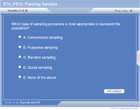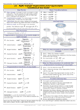How to Learn in 24 Hours?The Rapid Learning Movie

 Need Help? M-F: 9am-5pm(PST): Toll-Free: (877) RAPID-10 US Direct: (714) 692-2900 Int'l: 001-714-692-2900 24/7 Online Technical Support: The Rapid Support Center Secure Online Order:Need Proof? Testimonials by Our Users

 Rapid Learning Courses: MCAT in 24 Hours (2015-16) USMLE in 24 Hours (Boards) Chemistry in 24 Hours Biology in 24 Hours Physics in 24 Hours Mathematics in 24 Hours Psychology in 24 Hours SAT in 24 Hours ACT in 24 Hours AP in 24 Hours CLEP in 24 Hours DAT in 24 Hours (Dental) OAT in 24 Hours (Optometry) PCAT in 24 Hours (Pharmacy) Nursing Entrance Exams Certification in 24 Hours eBook - Survival Kits Audiobooks (MP3)

 Tell-A-Friend: Have friends taking science and math courses too? Tell them about our rapid learning system.Home »  Mathematics »  Introductory Statistics

Planning Samples and Experiments

Topic Review on "Title":
• Data: Observations (such as measurements, genders, Nutritional status) that have been collected.
• An element is the unit from or about whom survey information is collected
• Population: The total of all potential elements to whom survey results are to be generalized is called a population
• Statistics: A collection of methods for planning experiments, obtaining data, and then organizing, summarizing, presenting, analyzing, interpreting, and drawing conclusions based on the data.
• Census: The collection of data from every member of the population.
• Sample: A sub-collection of elements drawn from a population.
• Types of sampling:
• Random sampling: Each member of the population has an equal and known chance of being selected.
• Systematic sampling: After the required sample size has been calculated, every Nth record is selected from a list of population members
• Stratified sampling: The population is divided into homogeneous subgroups (strata). Each stratum is then sampled individually.
• Cluster sampling: The total population is divided into these groups (or clusters), and a sample of the groups is selected. Then the required information is collected from the elements within each selected group.
• Convenience sampling: is used in exploratory research where the researcher is interested in getting an inexpensive approximation of the truth. The sample is selected as per convenience.
• Purposive Sampling: Subjects are selected because of some characteristic
• Errors/bias in Sampling: Sampling error & non sampling error
• Type I and Type II Errors
 True state Decision from sample Accept H0 Accept H1 Ho is true No error Type I error (a) H1 is rue Type II error (b) No error
• a is called the significance level  of the test
• b is called power of the test

Rapid Study Kit for "Title":
 Flash Movie Flash Game Flash Card Core Concept Tutorial Problem Solving Drill Review Cheat Sheet"Title" Tutorial Summary : This tutorial describes planning samples and experiments. By completing this tutorial, you will learn about the steps in planning samples and experiments, such as research, data survey, sampling method, sample size, hypothesis testing, statistical analysis. Different types of errors and hypothesis testing are also discussed in this chapter accompanied with illustrative examples.

 Tutorial Features: Specific Tutorial Features: This tutorial analyzes the steps of survey and types of survey, step by step. Formula for determining sample size is proposed. You will learn how to decide a proper sample size in carrying out a survey sampling. Series Features: Concept map showing inter-connections of new concepts in this tutorial and those previously introduced. Definition slides introduce terms as they are needed. Visual representation of concepts Animated examples—worked out step by step A concise summary is given at the conclusion of the tutorial.

 "Title" Topic List: Data Population Statistics Census Sample Types of sampling Random sampling Systematic sampling Stratified sampling Cluster sampling Convenience sampling Purposive Sampling Errors/bias in Sampling

See all 24 lessons in Introductory Statistics, including concept tutorials, problem drills and cheat sheets:
Teach Yourself Introductory Statistics Visually in 24 Hours